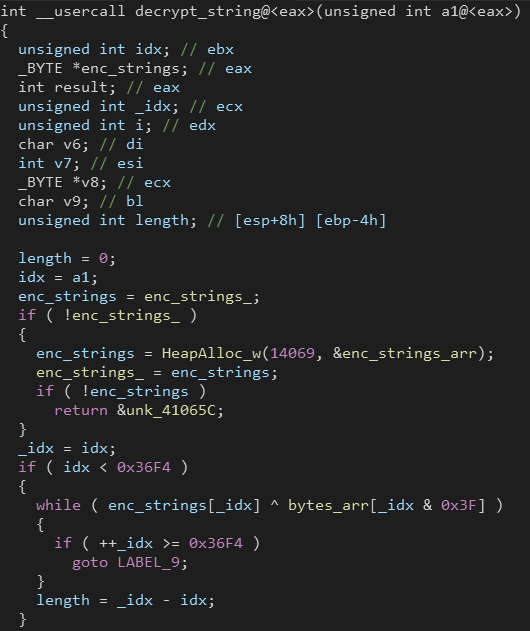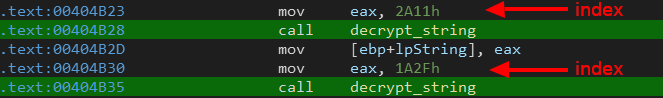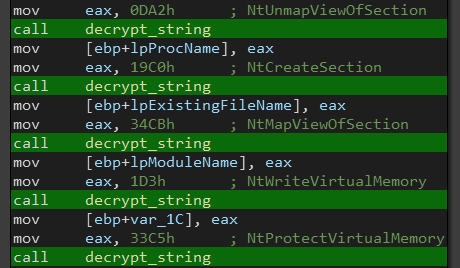# Qiling For Malware Analysis: Part 2

In the first part we talked about the basics of Qiling, you can find it here.

Now it’s time for some real world stuff, we will go through two scenarios where Qiling shines.

# Fetching KSLØT Dynamic Imports

Dynamic Imports or Dynamic API resolving is a common technique used by many malware samples to make static analysis harder. Instead of importing all needed APIs, the malware can store the APIs names or hashes then import them dynamically at runtime.

The most common way to do this is by using `LoadLibrary()` and `GetProcAddress()`, and that’s what we are KSLØT uses.

According to MSDN, the second argument to`GetProcAddress()` is the function name (`"lpProcName"`). So we can hook `GetProcAddress()` and dump the second argument each time it’s called.

Now you might be thinking, why don’t we just use a debugger and trace the execution flow of the malware ?

I can think of three problems about that approach:

• The malware might be implementing Anti-Debugging/Anti-Analysis tricks to waste your time
• The malware might run on a different architecture that you don’t have access to.
• You might want to automate the whole process (Scalability)

Let’s start writing the script.

``````from qiling import *
from qiling.const import *

# initialize emulator (x86_64 windows)
ql = Qiling(["kSLØT_Keylogger.dll"], "qiling/examples/rootfs/x8664_windows")
``````

The malware sample used here is distributed as a DLL file.

Similar to the main function in typical executables, DLLs have their `DllMain` function that is executed automatically when they are loaded into memory.

``````BOOL WINAPI DllMain(
HINSTANCE hinstDLL,  // handle to DLL module
DWORD fdwReason,     // reason for calling function
LPVOID lpReserved    // reserved
)
``````

As we can see, the function takes 3 arguments. The first one (`hinstDLL`) is a handle to the memory area where the DLL has been loaded. The second one stores a value that indicates the reason why the `DllMain` has been triggered. Read more here.

So to emulate the DLL properly, we need to set these arguments first (for x64 calling convention, paramerts are passed in `RCX`, `RDX`, `R8`, `R9`).

``````DLL_MAIN = 0x1800019a0     # Adress of DLLMain function
# hinstDLL
# fdwReason
ql.reg.rdx = 0x1           # DLL_PROCESS_DETACH
# lpvReserved
ql.reg.r8 = 0x0
``````

Next, we can use `set_api()` function to hook `GetProcAddress()` on exit.

``````#FARPROC GetProcAddress(
#  HMODULE hModule,
#  LPCSTR  lpProcName
#)
print("[*] Import: {}".format(params["lpProcName"]))

# disable logging
ql.filter = []
# start emulation
ql.run(begin=DLL_MAIN)
``````

Let’s see the results:

``````[+] Initiate stack address at 0x7ffffffde000
[+] PE entry point at 0x180006118
[*] Import: GetProcessImageFileNameW
[*] Import: GetForegroundWindow
[*] Import: GetWindowTextW
[*] Import: GetKeyboardState
...........
``````

Perfect! Knowing the imports of a malware sample can help in profiling it, BTW this malware is a keylogger.

# Decrypting QBot Strings

It’s common to see malware encrypting its strings to make the analysis process more challenging.

Recently I was analyzing QBot which implements this same technique and it only decrypts required strings on demand.

In my analysis, I reverse engineered the decryption routine as it was simple. But imagine if it was a complicated algorithm with lots of mathematical operations and obfuscated instructions, that’s where Qiling comes in handy.As you can see, the decryption function takes one argument in `EAX` which is an index and the returns the required string decrypted.We can combine the power of Qiling and IDAPython to decrypt the strings and add them as IDA comments.

First we need to get all cross references to the decryption function and then extract the index (second operand) from the previous instruction (as shown above).

``````# start/end of the decryption function
DEC_START = 0x4065B7
DEC_END   = 0x406655

# xrefs to the decryption function
xrefs = idautils.CodeRefsTo(DEC_START, 0)
# indexes of requested strings to decrypt
indexes = {}

for x in xrefs:
# address of previous instruction where "eax" is set
# type of the second operand of "mov"
t = idc.get_operand_type(ea, 1)
# check if the second operand is an immediate (not dynamic value)
if t == idc.o_imm:
# get the index value (second operand)
idx = idc.get_operand_value(ea, 1)
indexes[ea] = idx
``````

Next we initialize Qiling emulator object and loop through collected indexes. At each iteration we set `EAX` to the index value and run the decryption function.

Finally we read the decrypted string from `EAX` (return value) and set it as IDA comment.

``````# initialize emulator (x86 windows)
ql = Qiling(["qbot.exe"], rootfs="qiling/examples/rootfs/x86_windows")

# loop through collected indexes
for ea, idx in indexes.items():
# set function parameter @eax
ql.reg.eax = idx
# run decryption function
ql.run(begin=0x4065B7, end=0x406654)
# set decrypted string as ida comment
``````

Reading a string from memory address is simply reading bytes one by one until we reach a null byte.

``````# read string from memory address
res = ""
while True:
# read one byte at a time
if c == '\x00':
break
res += c
return res
``````

Let’s see the results:Viola! we managed to decrypt most of the strings without reversing the decryption function.

# Conclusion

Qiling is a great project for malware analysis and binary emulation. Although it’s still new but it has lots of capabilities and a lot more to come.

Code snippets can be found on my Github.

Don’t forget to star the Project to support the devs :)

Categories:

Updated: